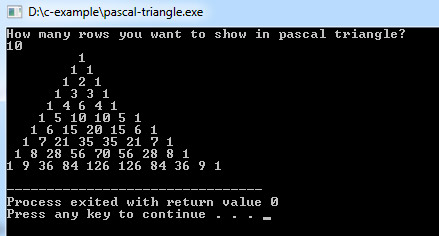## C Programming Examples Tutorial Index

This C program is used to print a pascal triangle.

Example:

``````#include<stdio.h>

long factorial(int);

int main()
{
int i, n, c;

printf("How many rows you want to show in pascal triangle?\n");

scanf("%d",&n);

for ( i = 0 ; i < n ; i++ )
{
for ( c = 0 ; c <= ( n - i - 2 ) ; c++ )
printf(" ");
for( c = 0 ; c <= i ; c++ )
printf("%ld ",factorial(i)/(factorial(c)*factorial(i-c)));
printf("\n");
}
return 0;
}

long factorial(int n)
{
int c;
long result = 1;

for( c = 1 ; c <= n ; c++ )
result = result*c;
return ( result );
}``````

Program Output:Explanation:

This program will create a pattern which consists of the Pascal triangle. So first of all, you have to include the stdio header file using the "include" preceding by # which tells that the header file needs to be process before compilation, hence named preprocessor directive. A long type user defined function prototype name - "factorial()" is being taken with parameter type as integer.

Then you have to define the main() function and it has been declared as integer so by default it returns integer. Inside the main() function you have to declare three integer type variable name - 'i', 'n' and 'c'. Then a printf() function is used which will prints the message - "How many rows you want to show in pascal triangle?" Then the scanf() function is used to fetch the data from the user and store it in 'n'.

After that a for-loop has to be implemented where counter variable I wil start from 0 till n-1. Inside this for loop there will be another for loop where its counter variable 'c' will start from 0 till (n-i-2). The statement printf(" ") wll place blank gap and another for is used which will calculate and display the value of factorial(i)/ (factorial(c)*factorial(i-c)). The next printf("\n") statement is used to go to the next line. The return 0; statement is used to return an integer type value back to main().

Now, within the factorial() function definition, an integer variable 'c' is declared and another variable name result of type long is also declared and initialized with value '1'. Now a for loop is implemented where counter variable 'c' starts from 1 till n and accordingly the value of result*c gets stored in result and gets returned.